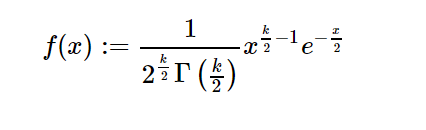# sympy.stats.ChiSquared() in python

• Last Updated : 05 Jun, 2020

With the help of `sympy.stats.ChiSquared()` method, we can get the continuous random variable representing the chi-squared distribution.Syntax : `sympy.stats.ChiSquared(name, k)`
Return : Return the continuous random variable.

Example #1 :
In this example we can see that by using `sympy.stats.ChiSquared()` method, we are able to get the continuous random variable which represents the chi-squared distribution by using this method.

 `# Import sympy and chisquared``from` `sympy.stats ``import` `ChiSquared, density``from` `sympy ``import` `Symbol`` ` `k ``=` `Symbol(``"k"``, integer ``=` `True``, positive ``=` `True``)``z ``=` `Symbol(``"z"``)`` ` `# Using sympy.stats.ChiSquared() method``X ``=` `ChiSquared(``"x"``, k)``gfg ``=` `density(X)(z)`` ` `pprint(gfg)`

Output :

-k k -z
— – – 1 —
2 2 2
2 *z *e
—————-
/k\
Gamma|-|
\2/

Example #2 :

 `# Import sympy and chisquared``from` `sympy.stats ``import` `ChiSquared, density``from` `sympy ``import` `Symbol`` ` `k ``=` `3``z ``=` `2`` ` `# Using sympy.stats.ChiSquared() method``X ``=` `ChiSquared(``"x"``, k)``gfg ``=` `density(X)(z)`` ` `pprint(gfg)`

Output :

-1
e
——
____
\/ pi

My Personal Notes arrow_drop_up